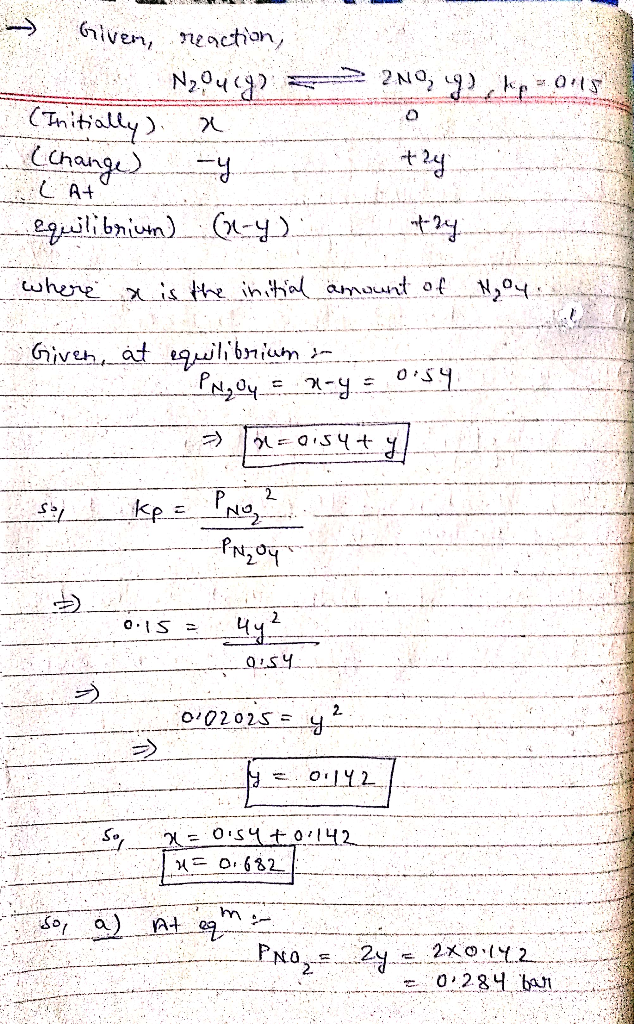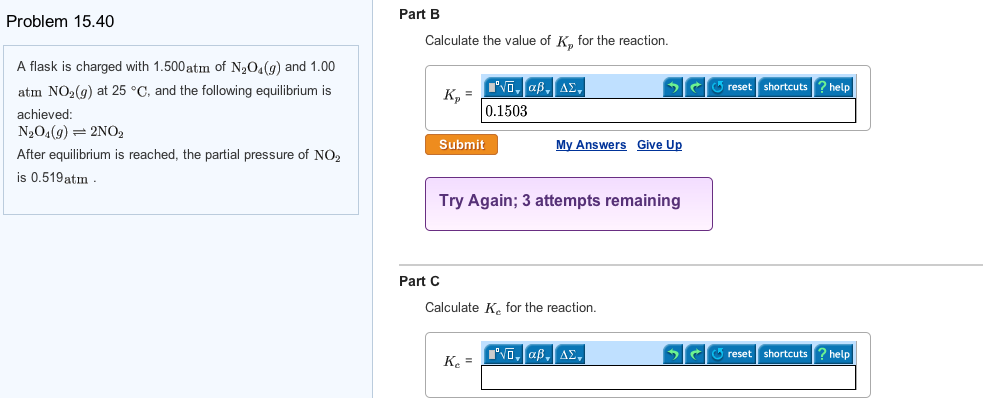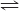Question

Kp for the reaction: N2O4(g)<======> 2NO2(g) in 25C is 0.15.

A certain amount of N2O4(g) is being inserted into a container in 25C

and at equilibrium, the pressure was 0.54 bar.

a. what is the partial pressure of each of the system's components at equilibrium?

**show detailed calculations in salving the two equations that you will receive.

(answers should be : p=0.43, x=0.11

so that: Peq(N2O4)= 0.32bar

And Peq(NO2)=0.22bar)#### Earn Coins

Coins can be redeemed for fabulous gifts.

Similar Homework Help Questions
• ### The total pressure for a mixture of N2O4 and NO2 is 0.15 atm. If Kp =...

The total pressure for a mixture of N2O4 and NO2 is 0.15 atm. If Kp = 7.1 (at 25 degree celsius), calculate the partial pressure of each gas in the mixture 2NO2(g) <---> N2O4(g)

• ### Consider the reaction:             N2O4(g) ⇄ 2NO2(g)   Kp = 80 In which of the following systems will...

Consider the reaction:             N2O4(g) ⇄ 2NO2(g)   Kp = 80 In which of the following systems will the reaction proceed in a direction to use up some of the NO2 (from right to left in the above equation).                         Partial Pressure N2O4 Partial pressure NO2 X 0.0020 atm 0.400 atm Y 0.0040 atm 0.800 atm Z 0.0040 atm 0.300 atm A. Y and Z only B. Z only C. Y only D. X, Y, and Z E. X and Y only...

• ### Question text Calculate the equilibrium constants, KpKp and KcKc for the equilibrium reaction N2O4(g)⇄2NO2(g)N2O4(g)⇄2NO2(g) at 298...

Question text Calculate the equilibrium constants, KpKp and KcKc for the equilibrium reaction N2O4(g)⇄2NO2(g)N2O4(g)⇄2NO2(g) at 298 K. N2O4(g)N2O4(g) NO2(g)NO2(g) S0S0 (J/K/mol) 304.29 240.06 ΔfH0ΔfH0    (kJ/mol) 9.16 33.18 Select one or more: A. Kp=9.23Kp=9.23 , Kc=12.3Kc=12.3 B. Kp=0.563Kp=0.563 , Kc=0.33Kc=0.33 C. Kp=0.144Kp=0.144 , Kc=0.0058Kc=0.0058 D. Kp=0.355Kp=0.355 , Kc=1.23

• ### At a particular temperature, Kp = 0.260 for the reaction N2O4 ---> <--- 2NO2 1. A...

At a particular temperature, Kp = 0.260 for the reaction N2O4 ---> <--- 2NO2 1. A flask containing only N2O4(g) at an initial pressure of 4.20 atm is allowed to reach equilibrium. Calculate the total pressure in this flask at equilibrium. 2. With no change in the amount of material in the flask, the volume of the container in question is decreased to 0.400 times the original volume. Assuming constant temperature, calculate the (new) total pressure, at equilibrium.

• ### Consider the following reaction. 2NO2(g)⇌N2O4(g) When the system is at equilibrium, it contains NO2 at a...

Consider the following reaction. 2NO2(g)⇌N2O4(g) When the system is at equilibrium, it contains NO2 at a pressure of 0.870 atm, and N2O4 at a pressure of 0.0757 atm. The volume of the container is then reduced to half its original volume. What is the pressure of each gas after equilibrium is reestablished?

• ### Nitrogen dioxide dimerizes according to the following reaction: 2NO2(g)⇌N2O4(g) Kp=6.7at298K A 2.35-L container contains 0.057 mol...

Nitrogen dioxide dimerizes according to the following reaction: 2NO2(g)⇌N2O4(g) Kp=6.7at298K A 2.35-L container contains 0.057 mol of NO2 and 0.084 molof N2O4at298K. Calculate Kc for the reaction. Express the equilibrium constant with respect to concentration to three significant figures. Calculate Q for the reaction. Express the reaction quotient to three significant figures.

• ### A flask is charged with 1.500atm of N2O4(g) and 1.00 atm NO2(g) at 25 degree C,...A flask is charged with 1.500atm of N2O4(g) and 1.00 atm NO2(g) at 25 degree C, and the following equilibrium is achieved: N2O4(g) 2NO2 After equilibrium is reached, the partial pressure of NO2 is 0.519atm. Calculate the value of Kp for the reaction. Calculate Kc for the reaction.

• ### Dinitrogen tetroxide decomposes to nitrogen dioxide: N2O4(g)→2NO2(g) ΔrH∘=55.3kJmol−1 At 298 K, a reaction vessel initially contains...

Dinitrogen tetroxide decomposes to nitrogen dioxide: N2O4(g)→2NO2(g) ΔrH∘=55.3kJmol−1 At 298 K, a reaction vessel initially contains 0.100 bar of N2O4. When equilibrium is reached, 58% of the N2O4 has decomposed to NO2. What percentage of N2O4 decomposes at 369 K? Assume that the initial pressure of N2O4 is the same (0.100 bar)

• ### Dinitrogen tetroxide decomposes to nitrogen dioxide: N2O4(g)→2NO2(g) ΔrH∘=55.3kJmol−1 At 298 K, a reaction vessel initially contains...

Dinitrogen tetroxide decomposes to nitrogen dioxide: N2O4(g)→2NO2(g) ΔrH∘=55.3kJmol−1 At 298 K, a reaction vessel initially contains 0.100 bar of N2O4. When equilibrium is reached, 58% of the N2O4 has decomposed to NO2. What percentage of N2O4 decomposes at 389 K? Assume that the initial pressure of N2O4 is the same (0.100 bar). Please use bar and not atm.

• ### A flask is charged with 1.700 atm of N2O4(g) and 1.050 atm NO2(g) at 25°C. The equilibrium...A flask is charged with 1.700 atm ofN2O4(g) and 1.050 atm NO2(g) at 25°C.The equilibrium reaction is given in theequation below.N2O4(g) 2NO2(g)After equilibrium is reached, the partial pressure ofNO2 is 0.541 atm.(a) What is the equilibrium partial pressure ofN2O4? 1 atm(b) Calculate the value of Kp for thereaction. 2